# kinetics (Lec 1).pptx

12 de Mar de 2023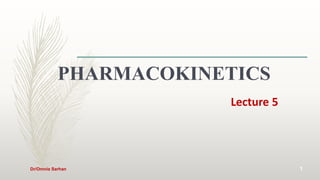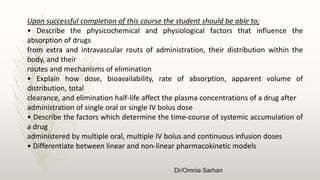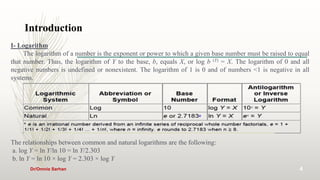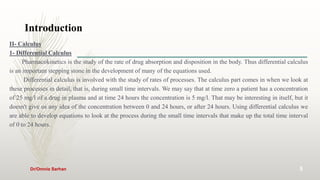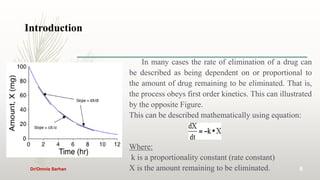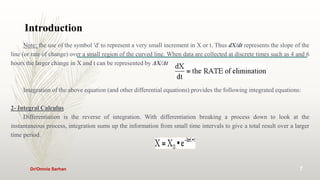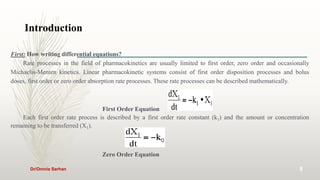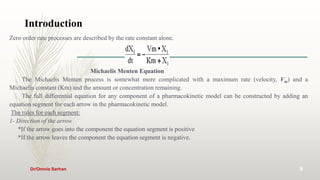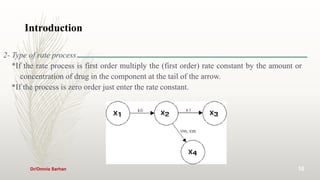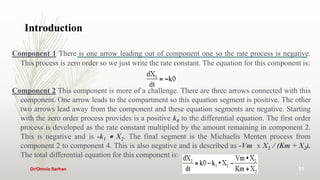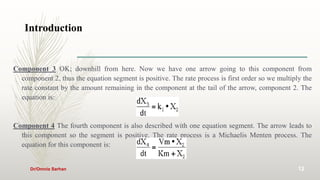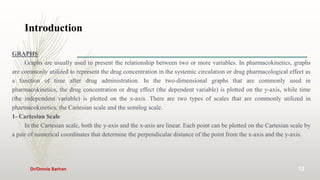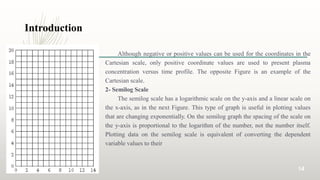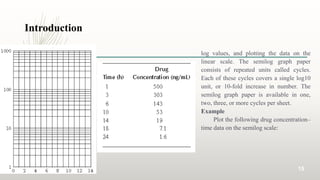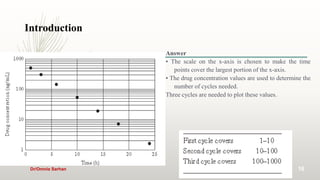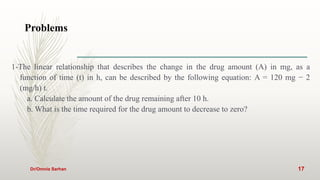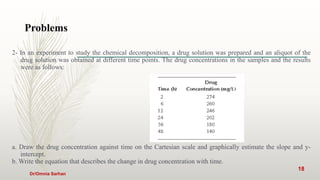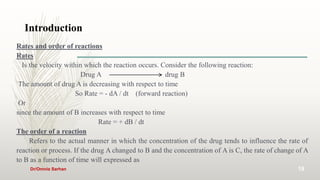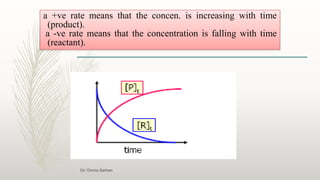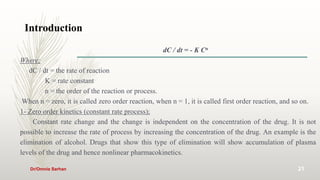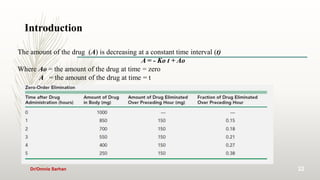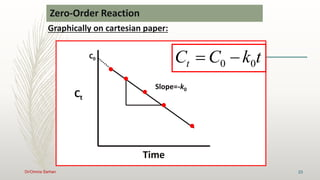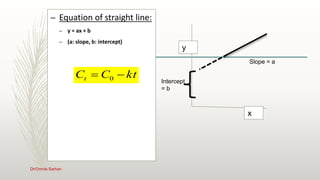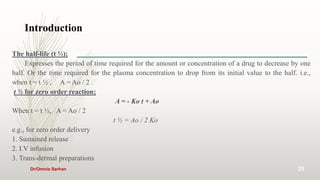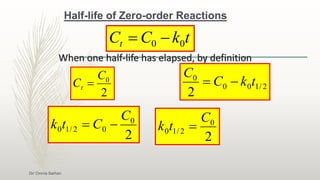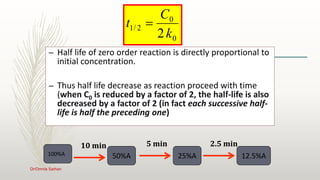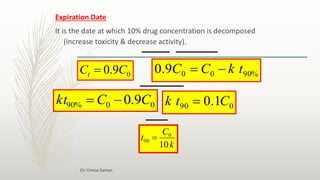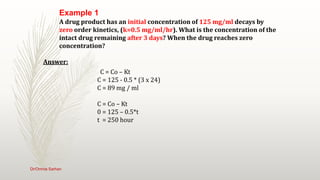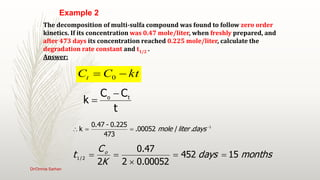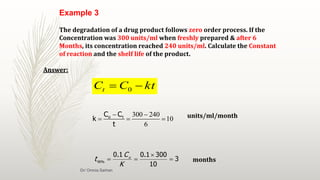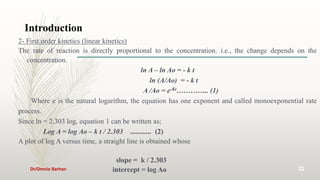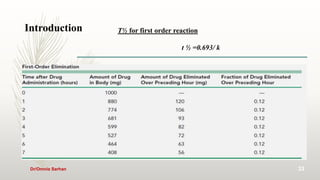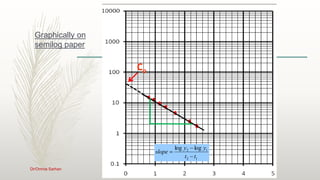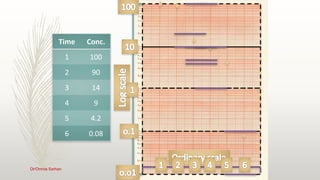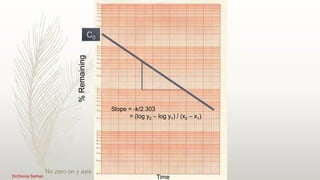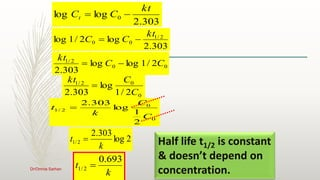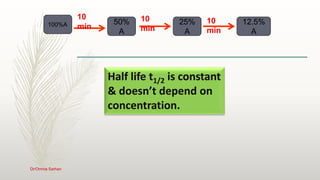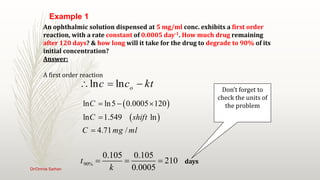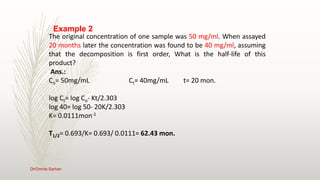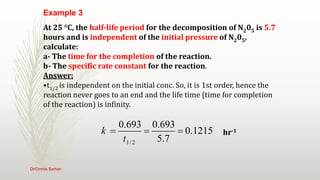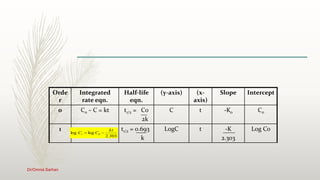1 de 43

### kinetics (Lec 1).pptx

• 2. Dr/Omnia Sarhan ‫الكليـــــــــــــة‬ ‫رؤيــــــــــــة‬ ‫الكليــــــــــــة‬ ‫رســـــــــــــــالة‬ ‫الريادة‬ ً ‫محليا‬ ً ‫واقليميا‬ ً ‫ودوليا‬ ‫لالرتقاء‬ ‫بصحة‬ ‫االنسان‬ ‫من‬ ‫خالل‬ ‫استخدا‬ ‫م‬ ‫االبتكارات‬ ‫فى‬ ‫مجال‬ ‫التعليم‬ ‫والبحوث‬ ‫العلمية‬ ‫والممارسات‬ ‫التطبيقية‬ . ‫توفير‬ ‫أفضل‬ ‫الممارسات‬ ‫في‬ ‫مجال‬ ‫التعليم‬ ‫والتعلم‬ ‫والتدريب‬ ‫وإستمرارية‬ ‫خ‬ ‫لق‬ ‫فرص‬ ‫للطالب‬ ‫والخريجين‬ ‫بإبتكار‬ ‫ونشر‬ ‫وتطبيق‬ ‫المعرفة‬ ‫الحديثة‬ ‫المرتكزة‬ ‫على‬ ‫البحوث‬ ‫والتطبيقات‬ ‫في‬ ‫العلوم‬ ‫الصيدلية‬ ‫واإلكلينيكية‬ ‫واإلجتماعية‬ ‫للنهوض‬ ‫بال‬ ‫صحة‬
• 3. Dr/Omnia Sarhan 3 Upon successful completion of this course the student should be able to; • Describe the physicochemical and physiological factors that influence the absorption of drugs from extra and intravascular routs of administration, their distribution within the body, and their routes and mechanisms of elimination • Explain how dose, bioavailability, rate of absorption, apparent volume of distribution, total clearance, and elimination half-life affect the plasma concentrations of a drug after administration of single oral or single IV bolus dose • Describe the factors which determine the time-course of systemic accumulation of a drug administered by multiple oral, multiple IV bolus and continuous infusion doses • Differentiate between linear and non-linear pharmacokinetic models
• 4. Introduction Dr/Omnia Sarhan 4 I- Logarithm The logarithm of a number is the exponent or power to which a given base number must be raised to equal that number. Thus, the logarithm of Y to the base, b, equals X, or log b (Y) = X. The logarithm of 0 and all negative numbers is undefined or nonexistent. The logarithm of 1 is 0 and of numbers <1 is negative in all systems. The relationships between common and natural logarithms are the following: a. log Y = ln Y/ln 10 = ln Y/2.303 b. ln Y = ln 10 × log Y = 2.303 × log Y
• 5. Introduction Dr/Omnia Sarhan 5 II- Calculus 1- Differential Calculus Pharmacokinetics is the study of the rate of drug absorption and disposition in the body. Thus differential calculus is an important stepping stone in the development of many of the equations used. Differential calculus is involved with the study of rates of processes. The calculus part comes in when we look at these processes in detail, that is, during small time intervals. We may say that at time zero a patient has a concentration of 25 mg/l of a drug in plasma and at time 24 hours the concentration is 5 mg/l. That may be interesting in itself, but it doesn't give us any idea of the concentration between 0 and 24 hours, or after 24 hours. Using differential calculus we are able to develop equations to look at the process during the small time intervals that make up the total time interval of 0 to 24 hours.
• 6. Introduction Dr/Omnia Sarhan 6 In many cases the rate of elimination of a drug can be described as being dependent on or proportional to the amount of drug remaining to be eliminated. That is, the process obeys first order kinetics. This can illustrated by the opposite Figure. This can be described mathematically using equation: Where: k is a proportionality constant (rate constant) X is the amount remaining to be eliminated.
• 7. Introduction Dr/Omnia Sarhan 7 Note: the use of the symbol 'd' to represent a very small increment in X or t. Thus dX/dt represents the slope of the line (or rate of change) over a small region of the curved line. When data are collected at discrete times such as 4 and 6 hours the larger change in X and t can be represented by ΔX/Δt Integration of the above equation (and other differential equations) provides the following integrated equations: 2- Integral Calculus Differentiation is the reverse of integration. With differentiation breaking a process down to look at the instantaneous process, integration sums up the information from small time intervals to give a total result over a larger time period.
• 8. Introduction Dr/Omnia Sarhan 8 First: How writing differential equations? Rate processes in the field of pharmacokinetics are usually limited to first order, zero order and occasionally Michaelis-Menten kinetics. Linear pharmacokinetic systems consist of first order disposition processes and bolus doses, first order or zero order absorption rate processes. These rate processes can be described mathematically. First Order Equation Each first order rate process is described by a first order rate constant (k1) and the amount or concentration remaining to be transferred (X1). Zero Order Equation
• 9. Introduction Dr/Omnia Sarhan 9 Zero order rate processes are described by the rate constant alone. Michaelis Menten Equation The Michaelis Menten process is somewhat more complicated with a maximum rate (velocity, Vm) and a Michaelis constant (Km) and the amount or concentration remaining. The full differential equation for any component of a pharmacokinetic model can be constructed by adding an equation segment for each arrow in the pharmacokinetic model. The rules for each segment: 1- Direction of the arrow *If the arrow goes into the component the equation segment is positive *If the arrow leaves the component the equation segment is negative.
• 10. Introduction Dr/Omnia Sarhan 10 2- Type of rate process *If the rate process is first order multiply the (first order) rate constant by the amount or concentration of drug in the component at the tail of the arrow. *If the process is zero order just enter the rate constant.
• 11. Introduction Dr/Omnia Sarhan 11 Component 1 There is one arrow leading out of component one so the rate process is negative. This process is zero order so we just write the rate constant. The equation for this component is: Component 2 This component is more of a challenge. There are three arrows connected with this component. One arrow leads to the compartment so this equation segment is positive. The other two arrows lead away from the component and these equation segments are negative. Starting with the zero order process provides is a positive k0 to the differential equation. The first order process is developed as the rate constant multiplied by the amount remaining in component 2. This is negative and is -k1  X2. The final segment is the Michaelis Menten process from component 2 to component 4. This is also negative and is described as -Vm x X2 / (Km + X2). The total differential equation for this component is:
• 12. Introduction Dr/Omnia Sarhan 12 Component 3 OK; downhill from here. Now we have one arrow going to this component from component 2, thus the equation segment is positive. The rate process is first order so we multiply the rate constant by the amount remaining in the component at the tail of the arrow, component 2. The equation is: Component 4 The fourth component is also described with one equation segment. The arrow leads to this component so the segment is positive. The rate process is a Michaelis Menten process. The equation for this component is:
• 13. Introduction Dr/Omnia Sarhan 13 GRAPHS Graphs are usually used to present the relationship between two or more variables. In pharmacokinetics, graphs are commonly utilized to represent the drug concentration in the systemic circulation or drug pharmacological effect as a function of time after drug administration. In the two-dimensional graphs that are commonly used in pharmacokinetics, the drug concentration or drug effect (the dependent variable) is plotted on the y-axis, while time (the independent variable) is plotted on the x-axis. There are two types of scales that are commonly utilized in pharmacokinetics, the Cartesian scale and the semilog scale. 1- Cartesian Scale In the Cartesian scale, both the y-axis and the x-axis are linear. Each point can be plotted on the Cartesian scale by a pair of numerical coordinates that determine the perpendicular distance of the point from the x-axis and the y-axis.
• 14. Introduction Dr/Omnia Sarhan 14 Although negative or positive values can be used for the coordinates in the Cartesian scale, only positive coordinate values are used to present plasma concentration versus time profile. The opposite Figure is an example of the Cartesian scale. 2- Semilog Scale The semilog scale has a logarithmic scale on the y-axis and a linear scale on the x-axis, as in the next Figure. This type of graph is useful in plotting values that are changing exponentially. On the semilog graph the spacing of the scale on the y-axis is proportional to the logarithm of the number, not the number itself. Plotting data on the semilog scale is equivalent of converting the dependent variable values to their
• 15. Introduction Dr/Omnia Sarhan 15 log values, and plotting the data on the linear scale. The semilog graph paper consists of repeated units called cycles. Each of these cycles covers a single log10 unit, or 10-fold increase in number. The semilog graph paper is available in one, two, three, or more cycles per sheet. Example Plot the following drug concentration– time data on the semilog scale:
• 16. Introduction Dr/Omnia Sarhan 16 Answer • The scale on the x-axis is chosen to make the time points cover the largest portion of the x-axis. • The drug concentration values are used to determine the number of cycles needed. Three cycles are needed to plot these values.
• 17. Problems Dr/Omnia Sarhan 17 1-The linear relationship that describes the change in the drug amount (A) in mg, as a function of time (t) in h, can be described by the following equation: A = 120 mg − 2 (mg/h) t. a. Calculate the amount of the drug remaining after 10 h. b. What is the time required for the drug amount to decrease to zero?
• 18. Problems Dr/Omnia Sarhan 18 2- In an experiment to study the chemical decomposition, a drug solution was prepared and an aliquot of the drug solution was obtained at different time points. The drug concentrations in the samples and the results were as follows: a. Draw the drug concentration against time on the Cartesian scale and graphically estimate the slope and y- intercept. b. Write the equation that describes the change in drug concentration with time.
• 19. Introduction 19 Rates and order of reactions Rates Is the velocity within which the reaction occurs. Consider the following reaction: Drug A drug B The amount of drug A is decreasing with respect to time So Rate = - dA / dt (forward reaction) Or since the amount of B increases with respect to time Rate = + dB / dt The order of a reaction Refers to the actual manner in which the concentration of the drug tends to influence the rate of reaction or process. If the drug A changed to B and the concentration of A is C, the rate of change of A to B as a function of time will expressed as Dr/Omnia Sarhan
• 20. a +ve rate means that the concen. is increasing with time (product). a -ve rate means that the concentration is falling with time (reactant). Dr/ Omnia Sarhan
• 21. Introduction 21 dC / dt = - K Cn Where; dC / dt = the rate of reaction K = rate constant n = the order of the reaction or process. When n = zero, it is called zero order reaction, when n = 1, it is called first order reaction, and so on. 1- Zero order kinetics (constant rate process); Constant rate change and the change is independent on the concentration of the drug. It is not possible to increase the rate of process by increasing the concentration of the drug. An example is the elimination of alcohol. Drugs that show this type of elimination will show accumulation of plasma levels of the drug and hence nonlinear pharmacokinetics. Dr/Omnia Sarhan
• 22. Introduction 22 The amount of the drug (A) is decreasing at a constant time interval (t) A = - Ko t + Ao Where Ao = the amount of the drug at time = zero A = the amount of the drug at time = t Dr/Omnia Sarhan
• 23. Zero-Order Reaction Graphically on cartesian paper: t k C Ct 0 0   23 Time Ct C0 Slope=-k0 t k C Ct 0 0   Dr/Omnia Sarhan
• 24. – Equation of straight line: – y = ax + b – (a: slope, b: intercept) x y Intercept = b Slope = a kt C Ct   0 24 Dr/Omnia Sarhan
• 25. Introduction 25 The half-life (t ½); Expresses the period of time required for the amount or concentration of a drug to decrease by one half. Or the time required for the plasma concentration to drop from its initial value to the half. i.e., when t = t ½ , A = Ao / 2 . t ½ for zero order reaction; A = - Ko t + Ao When t = t ½, A = Ao / 2 t ½ = Ao / 2 Ko e.g., for zero order delivery 1. Sustained release 2. I.V infusion 3. Trans-dermal preparations Dr/Omnia Sarhan
• 26. Half-life of Zero-order Reactions 0 0 2 / 1 2k C t  t k C Ct 0 0   When one half-life has elapsed, by definition 2 / 1 0 0 0 2 t k C C   2 0 C Ct  2 0 0 2 / 1 0 C C t k   2 0 2 / 1 0 C t k  Dr/ Omnia Sarhan
• 27. 0 0 2 / 1 2k C t  – Half life of zero order reaction is directly proportional to initial concentration. – Thus half life decrease as reaction proceed with time (when C0 is reduced by a factor of 2, the half-life is also decreased by a factor of 2 (in fact each successive half- life is half the preceding one) 100%A 10 min 50%A 25%A 12.5%A 5 min 2.5 min 27 Dr/Omnia Sarhan
• 28. Expiration Date It is the date at which 10% drug concentration is decomposed (increase toxicity & decrease activity). k C t 10 0 90  % 90 0 0 9 . 0 t k C C   0 9 . 0 C Ct  0 0 % 90 9 . 0 C C kt   0 90 1 . 0 C t k  Dr/ Omnia Sarhan
• 29. A drug product has an initial concentration of 125 mg/ml decays by zero order kinetics, (k=0.5 mg/ml/hr). What is the concentration of the intact drug remaining after 3 days? When the drug reaches zero concentration? Answer: C = Co – Kt C = 125 - 0.5 * (3 x 24) C = 89 mg / ml C = Co – Kt 0 = 125 – 0.5*t t = 250 hour Example 1 29 Dr/Omnia Sarhan
• 30. t C C k t o   1 . / 00052 . 473 0.225 - 0.47 k     days liter mole months days K C t o 15 452 00052 . 0 2 47 . 0 2 2 / 1      The decomposition of multi-sulfa compound was found to follow zero order kinetics. If its concentration was 0.47 mole/liter, when freshly prepared, and after 473 days its concentration reached 0.225 mole/liter, calculate the degradation rate constant and t1/2 . Answer: Example 2 kt C Ct   0 30 Dr/Omnia Sarhan
• 31. 300 240 10 6      o t C C k t     90% 0.1 0.1 300 3 10 o C t K The degradation of a drug product follows zero order process. If the Concentration was 300 units/ml when freshly prepared & after 6 Months, its concentration reached 240 units/ml. Calculate the Constant of reaction and the shelf life of the product. Answer: units/ml/month months Example 3 kt C Ct   0 Dr/ Omnia Sarhan
• 32. Introduction 32 2- First order kinetics (linear kinetics) The rate of reaction is directly proportional to the concentration. i.e., the change depends on the concentration. ln A – ln Ao = - k t ln (A/Ao) = - k t A /Ao = e-Kt………….. (1) Where e is the natural logarithm, the equation has one exponent and called monoexponential rate process. Since ln = 2.303 log, equation 1 can be written as; Log A = log Ao – k t / 2.303 ............ (2) A plot of log A versus time, a straight line is obtained whose slope = k / 2.303 intercept = log Ao Dr/Omnia Sarhan
• 33. Introduction 33 T½ for first order reaction t ½ =0.693/ k Dr/Omnia Sarhan
• 34. Graphically on semilog paper Co 1 2 1 2 log log t t y y slope    34 Dr/Omnia Sarhan
• 36. % Remaining Time C0 Slope = -k/2.303 = (log y2 – log y1) / (x2 – x1) No zero on y axis 36 Dr/Omnia Sarhan
• 37. k t 693 . 0 2 / 1  Half life t1/2 is constant & doesn’t depend on concentration. 2 log 303 . 2 2 / 1 k t  303 . 2 log log 0 kt C Ct   303 . 2 log 2 / 1 log 2 / 1 0 0 kt C C   0 0 2 / 1 2 1 log 303 . 2 C C k t  0 0 2 / 1 2 / 1 log log 303 . 2 C C kt   0 0 2 / 1 2 / 1 log 303 . 2 C C kt  37 Dr/Omnia Sarhan
• 38. 100%A 10 min 50% A 25% A 12.5% A 10 min 10 min Half life t1/2 is constant & doesn’t depend on concentration. 38 Dr/Omnia Sarhan
• 39. ln ln o c c kt        ln ln5 0.0005 120 ln 1.549 ln 4.71 / C C shift C mg ml      90% 0.105 0.105 210 0.0005 t k    Don’t forget to check the units of the problem An ophthalmic solution dispensed at 5 mg/ml conc. exhibits a first order reaction, with a rate constant of 0.0005 day-1. How much drug remaining after 120 days? & how long will it take for the drug to degrade to 90% of its initial concentration? Answer: A first order reaction days Example 1 39 Dr/Omnia Sarhan
• 40. The original concentration of one sample was 50 mg/ml. When assayed 20 months later the concentration was found to be 40 mg/ml, assuming that the decomposition is first order, What is the half-life of this product? Ans.: Co= 50mg/mL Ct= 40mg/mL t= 20 mon. log Ct= log Co- Kt/2.303 log 40= log 50- 20K/2.303 K= 0.0111mon-1 T1/2= 0.693/K= 0.693/ 0.0111= 62.43 mon. Example 2 40 Dr/Omnia Sarhan
• 41. 1/ 2 0.693 0.693 0.1215 5.7 k t    At 25 °C, the half-life period for the decomposition of N205 is 5.7 hours and is independent of the initial pressure of N205, calculate: a- The time for the completion of the reaction. b- The specific rate constant for the reaction. Answer: •t1/2 is independent on the initial conc. So, it is 1st order, hence the reaction never goes to an end and the life time (time for completion of the reaction) is infinity. hr-1 Example 3 41 Dr/Omnia Sarhan
• 42. Intercept Slope (x- axis) (y-axis) Half-life eqn. Integrated rate eqn. Orde r Co -Ko t C t1/2 = Co 2k Co – C = kt 0 Log Co -K 2.303 t LogC t1/2 = 0.693 k 1 303 . 2 log log 0 kt C Ct   Dr/Omnia Sarhan